In a previous post, we discussed this rule:

Mutually Exclusive Rule for P(A or B)
Let A, B be mutually exclusive events. Then, P(A or B) = P(A) + P(B).

Here, we talk about why this rule makes sense. First, an example:

Example 1
In rolling a fair, six-sided die, what is the probability that you will get a 1 or a 4?

Solution
We know that rolling a 1 and rolling a 4 are mutually exclusive events, since it is impossible for them both to occur. We know that P(rolling a 1) = ⅙ and that P(rolling a 4) = ⅙. Thus, by our above rule, P(Rolling a 1 or rolling a 4) = ⅙ + ⅙ = ⅓.

We may also consider the different possible outcomes that are relevant here: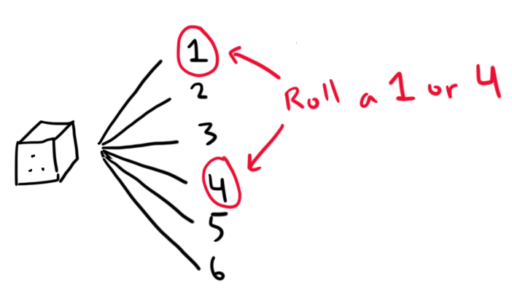So we roll a 1 or 4 in 2 of the six possible outcomes. Thus, the probability of doing so is just $\frac{2}{6} = \frac{1}{3}.$

In looking at some other examples, we may see why this more general rule makes sense:

General Rule for P(A or B)
Let A, B be two events. Then, P(A or B) = P(A) + P(B) - P(A and B).

Again, imagine we are rolling our favorite fair six-sided die. We are considering two events: A and B. We know that A occurs in three of the possible outcomes and B occurs in three of the possible outcomes. Can we determine P(A or B)? Now, this rule may seem a little arbitrary at first, but let’s see if we can see why it is true.

The answer is no. To see why, let’s consider two possible cases:

-       A: I roll an even number

-       B: I roll an odd number

Here, A occurs in three possible outcomes (2, 4, 6) and B occurs in three possible outcomes (1, 3, 5). And, in this case, P(A or B) = 1.

We can calculate this by considering how many of our outcomes satisfy A or B. So, for example, suppose we roll a 1. Then, “A or B” is true, since 1 is an odd number (so it is true that “I roll an odd number or I roll an even number”). Now suppose we roll a 2. Again, “A or B” is true, since 2 is an even number. Since all the numbers 1 through 6 are odd or even, we conclude that “A or B” will be true for all six outcomes. So, P(A or B) = 6/6 = 1.

But now consider this case:

-       C: I roll an even number

-       D: I roll a prime number

Again, C occurs in three possible outcomes (2, 4, 6) and D occurs in three possible outcomes (2, 3, 5). Now, in how many cases will “A or B” be true? Well let’s go through the cases. Suppose I roll a 1. Now, 1 is not even and it is not a prime number. So “C or D” will not be true if I roll a 1. Now consider 2; it is even, so “C or D” will be true if I roll a 2. And 3 is a prime number, 4 is even, 5 is prime, and 6 is even; so “C or D” will be true for all of those numbers.

Thus, P(A or B) = 5/6.

Thus, even when we know that A occurs in three of the possible outcomes and B occurs in three of the possible outcomes, we cannot determine P(A or B). (We are only able to do this if we get the additional information that A and B are mutually exclusive.)

To see why this is true, let’s picture each of the different outcomes as corresponding to some box.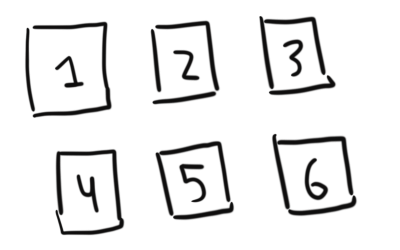Now, remember that for “A or B” to be true, we only need one of the two to be true. Let’s look at which boxes make A true.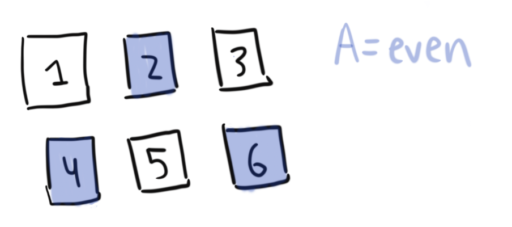And let’s now add which boxes make B true: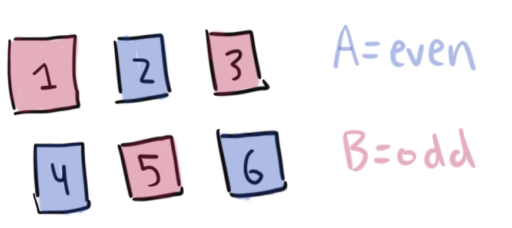So, the boxes that make “A or B” true will be all of the boxes.

But now let’s look at C and D. So, the boxes that make C true will be: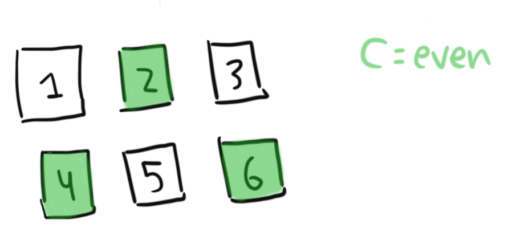And the boxes that make D true will be: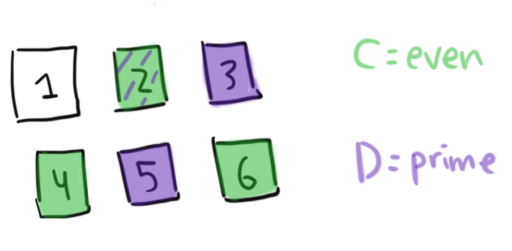Thus, the boxes that make “C or D” true will be only 5 of the 6 boxes. Looking at it this way, it becomes clear what went wrong: C and D overlap on one of the boxes. So we are “double-counting” the square 2. C counts it and D counts it. In some sense, we are wasting one of our boxes on 2.

That’s why our formula for calculating P(A or B) looks the way it does. First, we add the boxes where A is true. Second, we add the boxes where B is true.

But, now we’ve double-counted the boxes where A and B are both true – we added them once as part of A, and another time as part of B! That’s why our formula then has us subtract P(A and B). That way, we are cancelling out this double-counting.

So, to re-iterate, the formula for disjunctive probabilities is:

P(A or B) = P(A) + P(B) – P(A and B).

And from this general formula, we can derive the formula for Mutually Exclusive Disjunctions. So you only really need to learn this formula. How do we derive it?

Well, when A and B are mutually exclusive, we know that P(A and B) = 0. That’s just what it means to be mutually exclusive! Thus,

P(A or B) = P(A) + P(B) – P(A and B) = P(A) + P(B) – 0 = P(A) + P(B)

which was exactly our formula.